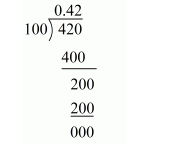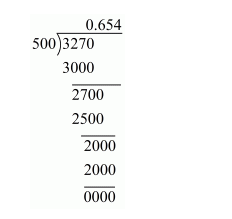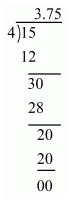# Express the following rational numbers as decimals:

Question:

Express the following rational numbers as decimals:

(i) $\frac{42}{100}$

(ii) $\frac{327}{500}$

(iii) $\frac{15}{4}$

Solution:

(i) Given rational number is $\frac{42}{100}$

Now we have to express this rational number into decimal form. So we will use long division method as below.Hence, $\frac{42}{100}=0.42$

(ii) Given rational number is $\frac{327}{500}$

Now we have to express this rational number into decimal form. So we will use long division method as below.Hence, $\frac{327}{500}=0.654$

(iii) Given rational number is $\frac{15}{4}$

Now we have to express this rational number into decimal form. So we will use long division method as below.Hence, $\frac{15}{4}=3.75$